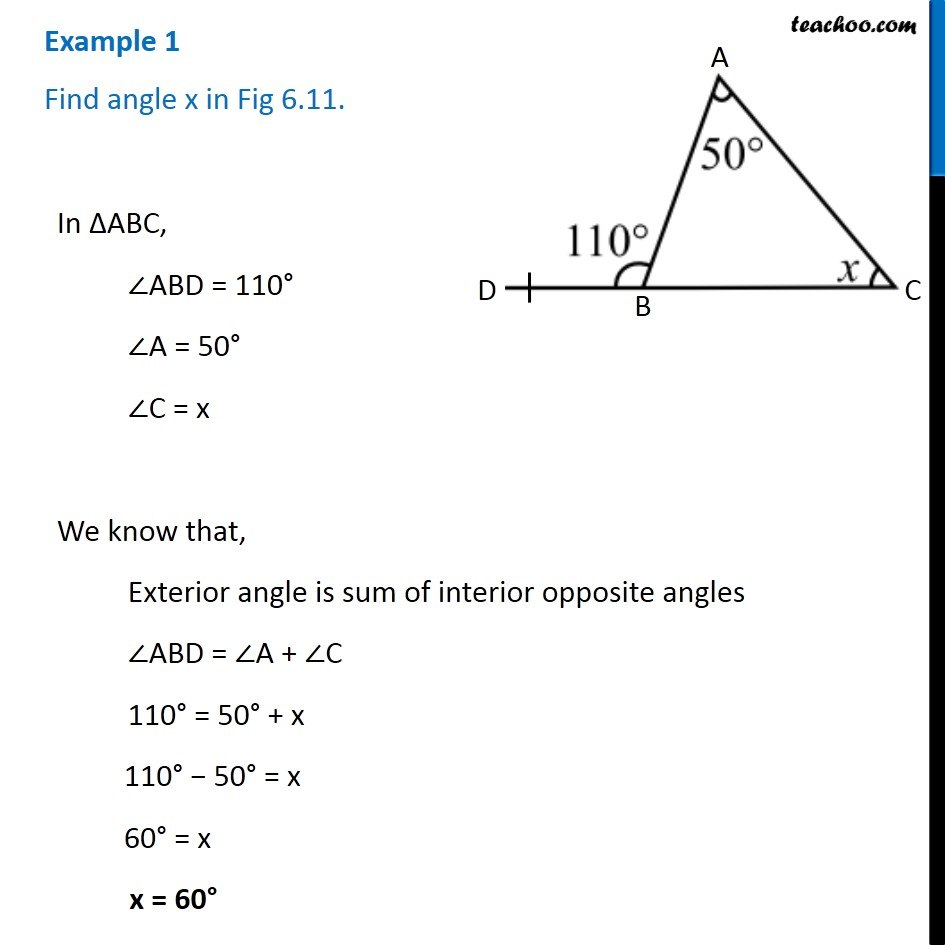1. Chapter 6 Class 7 Triangle and its Properties
2. Concept wise
3. Exterior angles of a triangle

Transcript

Example 1 - Chapter 6 Class 7 Triangle and its Properties - NCERT Book Find angle x in Fig 6.11. In ∆ABC, ∠ABD = 110° ∠A = 50° ∠C = x We know that, Exterior angle is sum of interior opposite angles ∠ABD = ∠A + ∠C 110° = 50° + x 110° − 50° = x 60° = x x = 60°